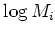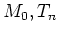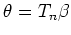Previous: 4.3 Choice of Residual Up: 4. Tuning of the Next: 4.5 Initial Values and

# 4.4 Linesearch

Physically the even moments represent positive quantities. The same property should be valid in the model. It was observed that the emergence of negative concentrations during a simulation run almost always resulted in non-convergence of the solver.

An obvious idea to avoid negative concentrations is to write the equations in terms of the logarithm of the even moments. Then this problem is eliminated. However, for the discretization the use of logarithmic variables is of disadvantage.

The dilemma can be solved in a similar way in which we tackled the problem of the formulation of the residual function. Again we use linear variables for calculation of the Jacobian. However, when doing the linesearch we use logarithmic variables.

The linesearch in the space of linear variables proceeds from the origin along a straight line. Linesearch in the logarithmic quantities starts from the same origin, but now we move along a straight line in the space of logarithmic quantities. Both curves have the same tangent in the origin. Hence we can calculate the logarithmic direction from the linear direction using the chain rule for differentiation. The same trick can be used for any set of independent variables. The implementation in MINIMOS-NT usesand(4.7)

as its variables. In this way it is possible to evaluate the effects of a change of independent variables with a minimum amount of extra implementation effort.

Compared to linear linesearch the logarithmic linesearch has very favorable properties and enhances the robustness of the solver.